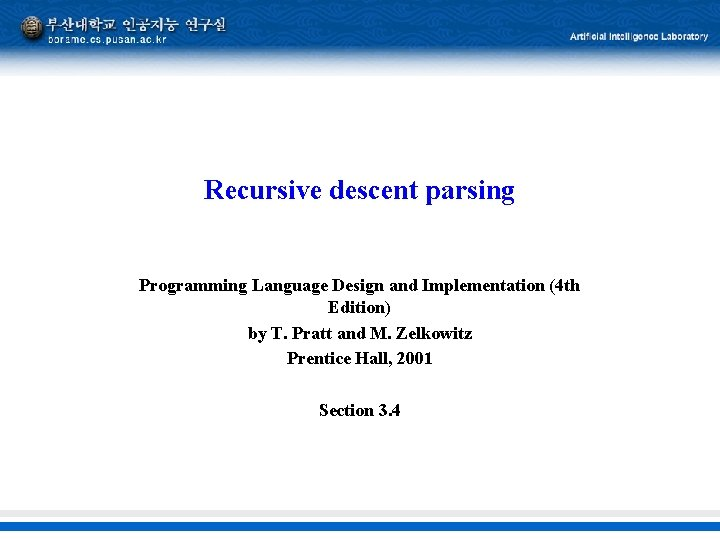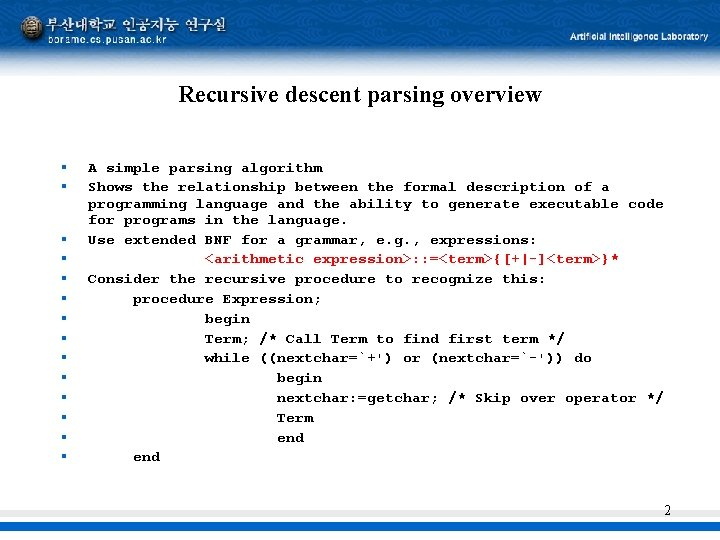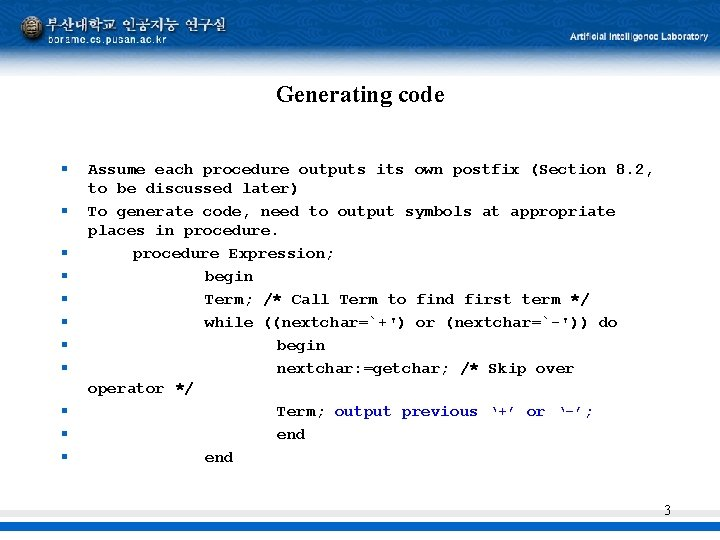# Recursive descent parsing Programming Language Design and Implementation

• Slides: 4Recursive descent parsing Programming Language Design and Implementation (4 th Edition) by T. Pratt and M. Zelkowitz Prentice Hall, 2001 Section 3. 4Recursive descent parsing overview § § § § A simple parsing algorithm Shows the relationship between the formal description of a programming language and the ability to generate executable code for programs in the language. Use extended BNF for a grammar, e. g. , expressions: <arithmetic expression>: : =<term>{[+|-]<term>}* Consider the recursive procedure to recognize this: procedure Expression; begin Term; /* Call Term to find first term */ while ((nextchar=`+') or (nextchar=`-')) do begin nextchar: =getchar; /* Skip over operator */ Term end 2Generating code § § § Assume each procedure outputs its own postfix (Section 8. 2, to be discussed later) To generate code, need to output symbols at appropriate places in procedure Expression; begin Term; /* Call Term to find first term */ while ((nextchar=`+') or (nextchar=`-')) do begin nextchar: =getchar; /* Skip over operator */ Term; output previous ‘+’ or ‘-’; end 3Generating code (continued) § § § § § Each non-terminal of grammar becomes a procedure. Each procedure outputs its own postfix. Examples: procedure Term; begin Primary; while ((nextchar=`*') or (nextchar=`/')) do begin nextchar: =getchar; /* Skip over operator */ Primary; output previous ‘*’ or ‘/’; end Procedure Identifier; begin if nextchar= letter output letter else error; nextchar=getchar; end Figure 3. 13 of text has complete parser for expressions. 4